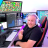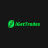# Looking for alterations on this Mobius Market internals script

B

#### BbenSD

##### New member
VIP
Hello everyone, so I've been using this Mobius script which is essentially market internals. It's pretty decent but I was wondering if anyone could help me do 1 thing pertaining to the script.

1. Make it usable on a tick chart

Here's the code, and thank you very much.

Code:
``````# Study Name: Quad_Momentum_Intraday

# Mobius

# V01.01.2012 Revised 03.12.2017

#hint: <b>This Study is for intraday trading only. The study uses Consolidated TICK, Advance Decline, Volume Breadth and Price for Trend Momentum. When all 4 agree the study plots a directional marker and colors the candles blue for ascending or yellow for descending price. The markers and candle color can be turned on and off with user inputs. The "n" input is for momentum length calculations.\n Trading Note: Look for the candle color or trend marker to "stop plotting" for indication trend may be slowing, possibly changing.\n Highest and best use is with a pivot study and a multiple contract Risk On / Risk off money management approach.

declare hide_on_daily;

input n = 8; #hint n: Length for Momentum calculations.

input CandleColor = yes;

input TrendMarkers = no;

input ShowData = yes;

input TickSymb = {default "\$TIKSP", "\$TICK", "\$TICK/Q"};

input VolSymb = {default "\$VOLSPD", "\$VOLQD", "\$VOLID"};

def tick = if IsNaN(close(TickSymb))

then tick

else close(TickSymb);

else close(VolSymb);

def price = close;

def Hrs = Floor(9.5 + SecondsFromTime(0930) / 60 / 60) - 1;

def Min = ((9.5 + SecondsFromTime(930) / 60 / 60) % 1) * 60;

def active = if SecondsFromTime(0930) >= 0 and

SecondsTillTime(1600) >= 0

then  1

else 0;

def lowestAD = if active and !active

else if active and

then Hrs

then Min

def highestAD = if active and !active

else if active and

then Hrs

then Min

def sumAD = if active and !active

else if active

def barLength = if active and !active

then 1

else if active

then barLength + 1

else barLength;

def CumTick = fold i = 0 to active

with p

while active

do CumTick + tick;

def CumTickMomo = (CumTick - Lowest(CumTick, n)) /

(Highest(CumTick, n) - Lowest(CumTick, n));

def PriceMomo = (price - Lowest(price, n)) /

(Highest(price, n) - Lowest(price, n));

plot QuadTrendUp = if CumTickMomo > .5 and

PriceMomo > .5

then low - TickSize()

else Double.NaN;

plot QuadTrendDn = if CumTickMomo < .5 and

PriceMomo < .5

then high + TickSize()

else Double.NaN;

def Range = Highest(high, n) - Lowest(low, n);

then CreateColor(25,49,250)

then Color.YELLOW

else Color.CURRENT);

"  Tick = " + tick +

"  || Breadth = " + Round(Breadth / 1000000, 2) + "m" +

"  || Range("+ n + ") = " + AsDollars(Round(Range / TickSize(), 0) * TickSize()),

then CreateColor(25,49,250)

then Color.YELLOW

else Color.WHITE);

•Looking for average price position line upper study indicators Questions 3Looking for Gap Up Scanner with EMA's Questions 1